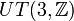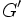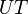# Powering-invariance does not satisfy lower central series condition in nilpotent group

This article gives the statement, and possibly proof, of a subgroup property (i.e., powering-invariant subgroup) satisfying a subgroup metaproperty (i.e., lower central series condition)
View all subgroup metaproperty satisfactions | View all subgroup metaproperty dissatisfactions |Get help on looking up metaproperty (dis)satisfactions for subgroup properties
Get more facts about powering-invariant subgroup |Get facts that use property satisfaction of powering-invariant subgroup | Get facts that use property satisfaction of powering-invariant subgroup|Get more facts about lower central series condition
This article gives the statement and possibly, proof, of a non-implication relation between two subgroup properties. That is, it states that every subgroup satisfying the first subgroup property (i.e., powering-invariant subgroup) need not satisfy the second subgroup property (i.e., LCS-powering-invariant subgroup)
View a complete list of subgroup property non-implications | View a complete list of subgroup property implications
Get more facts about powering-invariant subgroup|Get more facts about LCS-powering-invariant subgroup
EXPLORE EXAMPLES YOURSELF: View examples of subgroups satisfying property powering-invariant subgroup but not LCS-powering-invariant subgroup|View examples of subgroups satisfying property powering-invariant subgroup and LCS-powering-invariant subgroup

## Statement

It is possible to have a nilpotent group$G$, a powering-invariant subgroup$H$, and a positive integer$k$ such that$\gamma_k(H)$, the$k^{th}$ member of the lower central series of$H$, is not a powering-invariant subgroup of$\gamma_k(G)$, the$k^{th}$ member of the lower central series of$G$.

In fact, for any$k > 1$, we can construct an example that works for that value of$k$.

## Proof

### Proof for the derived subgroup (i.e.,$k = 2$)

Suppose$G$ is the direct product$UT(3,\mathbb{Q}) \times \mathbb{Z}$. The first direct factor here is unitriangular matrix group:UT(3,Q) and the second direct factor is the group of integers. Let$H$ be the subgroup$UT(3,\mathbb{Z})$ inside the first direct factor. Then:

•$H$ is a powering-invariant subgroup of$G$, because$G$ is not powered over any prime.
•$H'$ is not a powering-invariant subgroup of$G'$:$H'$ inside$G'$ looks like Z in Q, so it is not powering-invariant.

### Proof for arbitrary$k$

Take$G = UT(k+1,\mathbb{Q}) \times \mathbb{Z}$ and let$H = UT(k+1,\mathbb{Z})$ inside the first direct factor. Here,$UT$ denotes the unitriangular matrix group.